cancel
Showing results for
Did you mean:

Fabric is Generally Available. Browse Fabric Presentations. Work towards your Fabric certification with the Cloud Skills Challenge.## Calculate the Distance between Two Locations

Basic scenario:

When we want to calculate the distance between two locations, we need to know the coordinates of these two locations.

Here’s the sample data:We now have the coordinates of current locations and destinations, using below formula we could get the distance between these locations.

``````Distince (KM) =
12742 -- The diameter of the Earth (KM).
* ASIN (
-- Returns the arcsine, or inverse sine, of a number.
SQRT (
-- Returns the square root of a number.
POWER (
-- Returns the result of a number raised to a power.
SIN ( -- Returns the sine of the given angle.
'Table'[Current Lat] - 'Table'[Destination Lat] )
* PI () / 360,
2
) -- PI () Returns the value of Pi, 3.14159265358979, accurate to 15 digits.
+ COS (
-- Returns the cosine of the given angle.
'Table'[Current Lat] * PI () / 180
)
* COS ( 'Table'[Destination Lat] * PI () / 180 )
* POWER (
SIN ( 'Table'[Current Long] - 'Table'[Destination Long] )
* PI () / 360,
2
)
)
)``````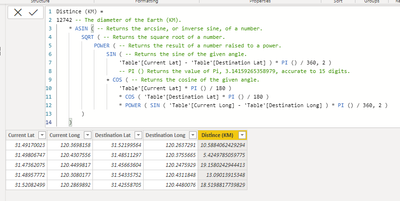Reference:

https://docs.microsoft.com/en-us/dax/sqrt-function-dax

https://docs.microsoft.com/en-us/dax/asin-function-dax

https://docs.microsoft.com/en-us/dax/power-function-dax

https://docs.microsoft.com/en-us/dax/sin-function-dax

https://docs.microsoft.com/en-us/dax/cos-function-dax

https://docs.microsoft.com/en-us/dax/pi-function-dax

Now we have two tables.

The Customer table contains the coordinates of some customers.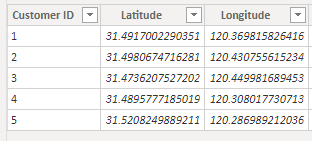And the Store table contains the coordinates of some stores.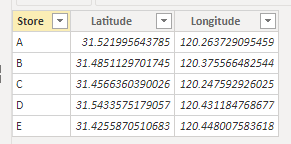By flexibly using the above formula of calculating distance, we can get the nearest store for each user and also get the distance from each user to their nearest store.

``````Nearest Store =
CALCULATE (
FIRSTNONBLANK ( Store[Store], 0 ),
TOPN (
1,
Store,
12742
* ASIN (
SQRT (
POWER ( SIN ( Customer[Latitude] - Store[Latitude] ) * PI () / 360, 2 )
+ COS ( Customer[Latitude] * PI () / 180 )
* COS ( Store[Latitude] * PI () / 180 )
* POWER ( SIN ( Customer[Longitude] - Store[Longitude] ) * PI () / 360, 2 )
)
), ASC
)
)``````
``````Distance to Nearest Store (KM) =
MINX (
Store,
12742
* ASIN (
SQRT (
POWER ( SIN ( Customer[Latitude] - Store[Latitude] ) * PI () / 360, 2 )
+ COS ( Customer[Latitude] * PI () / 180 )
* COS ( Store[Latitude] * PI () / 180 )
* POWER ( SIN ( Customer[Longitude] - Store[Longitude] ) * PI () / 360, 2 )
)
)
)``````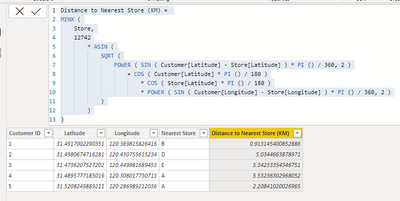Author: Jay Wang

Reviewer: Ula Huang, Kerry WangThis works for me if the distance to the nearest store = 0, but it pulls the wrong name for the rest of the stores even though the distance calculation looks correct. Any idea what I could be doing wrong? I am new to Power BI and DAX.

Hi all!

I have the table 'Lat_Long' with three columns: Branch_ID, Lat, Long and I want, when I select a branch, to calculate the distance between this branch and all the other.

Measure =

VAR Lat1 = SELECTEDVALUE('Lat_Long'[Lat])

VAR Lng1 = SELECTEDVALUE('Lat_Long'[Long])

VAR Lat2 = 'Lat_Long'[Lat]

VAR Lng2 = 'Lat_Long'[Long]

var P = DIVIDE( PI(), 180 )

var A = 0.5 - COS((Lat2-Lat1) * p)/2 + COS(Lat1 * p) * COS(lat2 * P) * (1-COS((Lng2- Lng1) * p))/2

var final = 12742 * ASIN((SQRT(A)))

return final

But, I see this error:

"A single value for column 'Lat' in table 'Lat_Long' cannot be determined. This can happen when a measure formula refers to a column that contains many values without specifying an aggregation such as min, max, count, or sum to get a single result."

Thank you!@stathar0s Try:

``````Measure =

VAR Lat1 = SELECTEDVALUE('Lat_Long'[Lat])

VAR Lng1 = SELECTEDVALUE('Lat_Long'[Long])

VAR Lat2 = MAX('Lat_Long'[Lat])

VAR Lng2 = MAX('Lat_Long'[Long])

var P = DIVIDE( PI(), 180 )

var A = 0.5 - COS((Lat2-Lat1) * p)/2 + COS(Lat1 * p) * COS(lat2 * P) * (1-COS((Lng2- Lng1) * p))/2

var final = 12742 * ASIN((SQRT(A)))

return final``````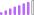Hi BI Community Team,

For 12742 is referred to the diameter of the earth (KM). If we consider and want to see it as Meters or Mile.

Thanks and Regards,@ADSL Miles is 7,922. Meters would be 12,742,000Hi BI Community Team,

Is it possible to show 02 locations on the map? It help us to know how far from current to destination, or easy to track as well.